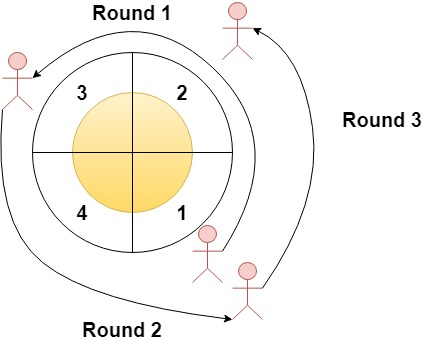# GeetCode Hub

Given an integer `n` and an integer array `rounds`. We have a circular track which consists of `n` sectors labeled from `1` to `n`. A marathon will be held on this track, the marathon consists of `m` rounds. The `ith` round starts at sector `rounds[i - 1]` and ends at sector `rounds[i]`. For example, round 1 starts at sector `rounds` and ends at sector `rounds`

Return an array of the most visited sectors sorted in ascending order.

Notice that you circulate the track in ascending order of sector numbers in the counter-clockwise direction (See the first example).

Example 1:```Input: n = 4, rounds = [1,3,1,2]
Output: [1,2]
Explanation: The marathon starts at sector 1. The order of the visited sectors is as follows:
1 --> 2 --> 3 (end of round 1) --> 4 --> 1 (end of round 2) --> 2 (end of round 3 and the marathon)
We can see that both sectors 1 and 2 are visited twice and they are the most visited sectors. Sectors 3 and 4 are visited only once.```

Example 2:

```Input: n = 2, rounds = [2,1,2,1,2,1,2,1,2]
Output: 
```

Example 3:

```Input: n = 7, rounds = [1,3,5,7]
Output: [1,2,3,4,5,6,7]
```

Constraints:

• `2 <= n <= 100`
• `1 <= m <= 100`
• `rounds.length == m + 1`
• `1 <= rounds[i] <= n`
• `rounds[i] != rounds[i + 1]` for `0 <= i < m`

class Solution { public List<Integer> mostVisited(int n, int[] rounds) { } }